Next: Pair Creation Up: Compton Scattering Previous: Compton Scattering

## Inverse Compton Scattering

When an electron is not at rest, but colliding with a soft photon, the recoiling photon gains its energy by the transfer from the incoming electrons. This process called Inverse Compton Scattering,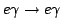, is a representative radiation in many astronomical environments that produces high energy X-ray/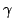-ray.

The colliding energy between a photon and an electron is often defined by the he Lorentz invariant center of mass (CM) energy squaredas defined by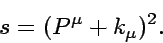(26)

Here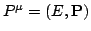and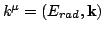are 4-momentum of the electron and the photon respectively.can be rewritten in the different system: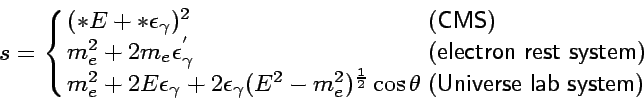(27)

Here natural unit is used i.e.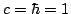. In this expression, the photon energy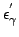in the electron rest system in Eq. 25 is written by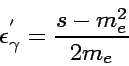(28)

in the Lorentz invariant form. The Lorentz transformation gives the relations between energies in the electron rest system and those in Universe lab system when incoming electron energy is by far higher than the target photon. Then one can calculate energy of the recoiling electron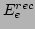as a function of incoming electron energy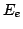in the lab system as(29)

where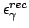is the recoiling energy of-ray in the Universe lab system. By using Eq. 29 we can modify the Kline-Nishina formula Eq. 25 to derive the energy differential cross section. We obtain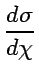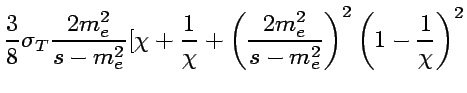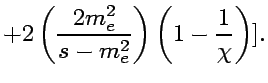(30)

Here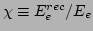is the dimensionless energy of the electron normalized by primary energy of incoming electron. The electron transfer most of its energy to the photon via the inverse Compton scattering as you see the cross section becomes larger in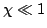regime in Eq. 30. Integrating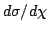fromto 1 (this range is given by Eq. 29 with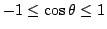), we obtain the total cross section: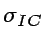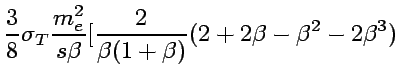(31)

where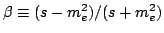is the velocity of the outgoing electron in the CMS.Next: Pair Creation Up: Compton Scattering Previous: Compton Scattering
Shigeru Yoshida
2002-07-18